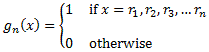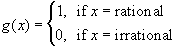#Interactive Real Analysis

Next | Previous | Glossary | Map

## 8.1. Pointwise Convergence

### Example 8.1.8 (c): Pointwise Convergence does not preserve Integrability

Find a pointwise convergent sequence of Riemann-integrable functions whose limit is not Riemann-integrable.

Let rn be the (countable) set of rational numbers inside the interval [0, 1], ordered in some way, and define the functionsandThen:

• The sequence gn converges pointwise to g
• Each gn has only finitely many points of discontinuity, thus each gn is Riemann integrable
• The limit function g is not Riemann integrable

Thus, pointwise convergence does not preserve Riemann-integrability.

Next | Previous | Glossary | Map